Mobile QR Code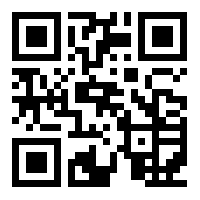1. (Division of Electronic, Information and Communications Eng.,Kangwon National University / Samcheok, Korea)

Impulsive noise, Range of influence, Information potential, Blind, DFE, Gaussian kernel

## 1. Introduction

Incomplete channel conditions are distorted by multipath fading and additive noise. These conditions induce intersymbol interference (ISI) that makes communication systems unreliable . For ISI cancellation, equalizer algorithms are used, and blind type algorithms are in great demand for communication systems where training symbols are not available. In in-vehicle signal transmissions  and underwater communications , impulsive noise exists as well as channel distortions and background Gaussian noise.

For cancellation of the residual ISI, decision feedback equalizer (DFE) algorithms are in demand, but there are some critical problems in the impulsive noise environment. Impulsive noise produces bursts of incorrect decisions that can cause error propagation in the DFE, and therefore, properties robust against impulsive noise without any additional techniques are highly recommended and can offer grounds for employing the decision feedback (DF) approach. For this purpose, a blind DFE cost function has been proposed based on an information theoretic learning method and the assumption that equi-probable symbol points are transmitted, and arelated decision feedback algorithm was presented for severely distorted channels contaminated with strong impulsive noise .

The information potential concept used in the information theoretic learning method was first introduced by Principe . The cost function in  contains two information potentials, and minimization of the cost function can be considered to produce harmonious interactions between the two forces; the spreading force on output sample pairs and the concentrating forceon pairs of symbol points and output samples.Based on this concept of harmonization of pushing and pulling forces, we propose a new version of our initial blind DFE by modifying kernel-sizesof the two information potentials in order to boost robustness against impulsive noiseas well as performance of ISI cancellation.

The inherent immunity of the blind algorithm to impulsive noise is analyzed in Section 2 and its decision feedback version is proposed in Section 3, aiming at robustness againstimpulsive noise and severe channel distortions. Section 4 reports simulation results and discussions. Finally, concluding remarks are presented in Section5.

## 2. Image Denoising Method

Given a set of $N$data samples, $\left\{x_{1},x_{2},\right.\left.\ldots ,x_{N}\right\}$, the PDF $f_{X}(x)$ based on the Parzen window method can be approximated by

##### (1)
$f_{X}(x)=\frac{1}{N}\sum _{i=1}^{N}G_{\sigma }(x-x_{i})$,

where $G_{\sigma }(\cdot )$is a zero-mean Gaussian kernel with standard deviation $\sigma$. When Shannon’s entropy is used along with this probability density estimation, an algorithm to estimate entropy becomes unrealistically complex . However, a much simpler form of entropy can be Reny’s quadratic entropy, $H_{\mathrm{Re}ny}(x)$ :

##### (2)
$H_{\mathrm{Re}ny}(x)=-\log (\frac{1}{N^{2}}\sum _{i=1}^{N}\sum _{j=1}^{N}G_{\sigma \sqrt{2}}(x_{j}-x_{i}))$.

The argument of the logarithm is defined as information potential that deals with the interaction of information particles that behave like physical ones .

##### (3)
$IP(x)=\frac{1}{N^{2}}\sum _{i=1}^{N}\sum _{j=1}^{N}G_{\sigma \sqrt{2}}(x_{j}-x_{i})$.

Then, the estimator for quadratic entropy expressed in (2) is a negative logarithm of the information potential:

##### (4)
$H_{\mathrm{Re}ny}(x)=-\log (IP(x))$.

From (4), we can see that minimizing or maximizing entropy is equivalent to maximizing or minimizing information potential, respectively.

Assuming that all the transmitted symbol points, $\left\{A_{1},A_{2},\ldots ,A_{M}\right\}$, are equally likely, cost function $C_{MED2}$ from  consists of two information potentials, $IP(d,y)$ and $IP(y,y)$, using the symbol points and a block of output samples, $\left\{y_{1},y_{2},\ldots ,y_{N}\right\}$, expressed as

##### (5)
$IP(d,y)=\frac{1}{M}\frac{1}{N}\sum _{m=1}^{M}\sum _{i=1}^{N}G_{\sigma }(A_{m}-y_{i})$,
##### (6)
$IP(y,y)=\frac{1}{N^{2}}\sum _{i=1}^{N}\sum _{j=1}^{N}G_{\sigma \sqrt{2}}(y_{j}-y_{i})$,

and

##### (7)
$C_{MED2}=IP(y,y)-2\cdot IP(d,y)$.

An excessively large value for $(y_{j}-y_{i})$ can usually be induced by impulsive noise, but the output of $G_{\sigma \sqrt{2}}(y_{j}-y_{i})$ becomes very small. And Gaussian kernel $G_{\sigma }(A_{m}-y_{i})$ in $IP(d,y)$ makes the cost function insensitive to large differences between symbol points and corrupted output samples. This inherent property of being immune to impulsive noise prevents incorrect decisions to some extent, so that error propagation can be avoided when a decision feedback structure is employed.

Decision feedback equalizer output $y_{k}$at sample time$k$comprises $P$ feed-forward filter weights, $\{w_{k,0}^{F},w_{k,1}^{F},w_{k,2}^{F},\ldots ,w_{k,P-1}^{F}\}$=$\mathbf{W}_{k}^{F}$, $Q$feedback filter weights, $\{w_{k,0}^{B},w_{k,1}^{B},w_{k,2}^{B},\ldots ,w_{k,Q-1}^{B}\}$=$\mathbf{W}_{k}^{B},$ and $\overset{\wedge }{\{d_{k-1}},\overset{\wedge }{d_{k-2}},\ldots ,$ $\overset{\wedge }{d_{k-Q-2}\}}$=$\hat{\mathbf{D}}_{k-1}$ previously decided symbols, described as follows:

##### (8)
$y_{k}=\sum _{p=0}^{P-1}w_{k,p}^{F}x_{k-p}+\sum _{q=0}^{Q-1}w_{k,q}^{B}\overset{\wedge }{d}_{k-q-1}$.

In the process for minimizingcost function (7), the blind DFE algorithm that adjusts the filter weights recursively is derived as seen in (9) and (10) . We refer to this as MED2 with DF in this paper, for the sake of convenience.

$w_{k+1,p}^{F}=w_{k,p}^{F}-$$\mu _{MED2}[\frac{1}{2N^{2}\sigma ^{2}}\sum _{i=k-N+1,}^{k}\sum _{j=k-N+1}^{k}(y_{j}-y_{i})$$ \cdot G_{\sigma \sqrt{2}}\left(y_{j}-y_{i}\right) \cdot\left(x_{i-p}-x_{j-p}\right) $$-\frac{2}{MN\sigma ^{2}}\sum _{i=k-N+1,}^{k}\sum _{m=1}^{M}(A_{m}-y_{i})\cdot G_{\sigma }(A_{m}-y_{i})\cdot x_{i-p}], ##### (10) w_{k+1,q}^{B}=w_{k,q}^{B}-$$\mu _{MED2}[\frac{1}{2N^{2}\sigma ^{2}}\sum _{i=k-N+1,}^{k}\sum _{j=k-N+1}^{k}(y_{j}-y_{i})$ $$\cdot G_{\sigma \sqrt{2}}\left(y_{j}-y_{i}\right) \cdot\left(\hat{d}_{i-1-q}-\hat{d}_{j-1-q}\right)$$ $-\frac{2}{MN\sigma ^{2}}\sum _{i=k-N+1,}^{k}\sum _{m=1}^{M}(A_{m}-y_{i})\cdot G_{\sigma }(A_{m}-y_{i})\cdot \overset{\wedge }{d}_{i-1-q}]$,

where $N\geq P$ and $N\geq Q$.

## 3. Modifying the IP Scope of Influence

For the information potential $IP(y,y)=$ $\frac{1}{N^{2}}\sum _{i=1}^{N}\sum _{j=1}^{N}G_{\sigma \sqrt{2}}(y_{j}-y_{i})$, which is the sum of all pairs of interactions, the term is built on the assumption that samples placed in the locations of $y_{i}$ and $y_{j}$ behave like charged particles in those locations. Similar to a repulsive electrostatic force, $G_{\sigma \sqrt{2}}(y_{j}-y_{i})$, the Gaussian kernel for samples $y_{i}$ and $y_{j}$ produces the extent of a repelling force between the two samples, which decays exponentially with the distance between the two. So that means increasing $IP(y,y)$ increases the repelling force among all the output samples. In effect, this operation brings them close together. Conversely, decreasing $IP(y,y)$ disperses the samples widely. The minimization of cost function (7) minimizes $IP(y,y)$ so that output samples become widely distributed searching for their own targets.

Turning our focus to the information potential scope of influence, we can see that kernel size $\sigma \sqrt{2}$ of $IP(y,y)$ plays a role in determining the range of influence. That is, a pair of samples located within distance $\sigma \sqrt{2}$ is under a strong potential, and the sample pair lets the gap distance between the pair increase, complying with minimization of $IP(y,y)$.

For instance, the scope of the $IP(y,y)$ influence exerted from $y_{i}$ to other samples is described in Fig. 1, where $A_{m}$ is the desired target symbol point, $A_{n}$ is another symbol point, and $y_{IM}$ (far from the other samples) is from impulsive noise. The two samples, $y_{2}$ and $y_{4}$, are within kernel size $\sigma \sqrt{2}$ of $G_{\sigma \sqrt{2}}(y_{j}-y_{i})$ in $IP(y,y)$, and they move away from$y_{i}$. As mentioned above, this spreading out movement is to search for their own targets. Samples $y_{1}$ and $y_{5}$ are outside the range so that they cannot move out searching for their own targets.

Fig. 1. Pushing force between$y_{i}$ and output samples induced from $IP(y,y)$.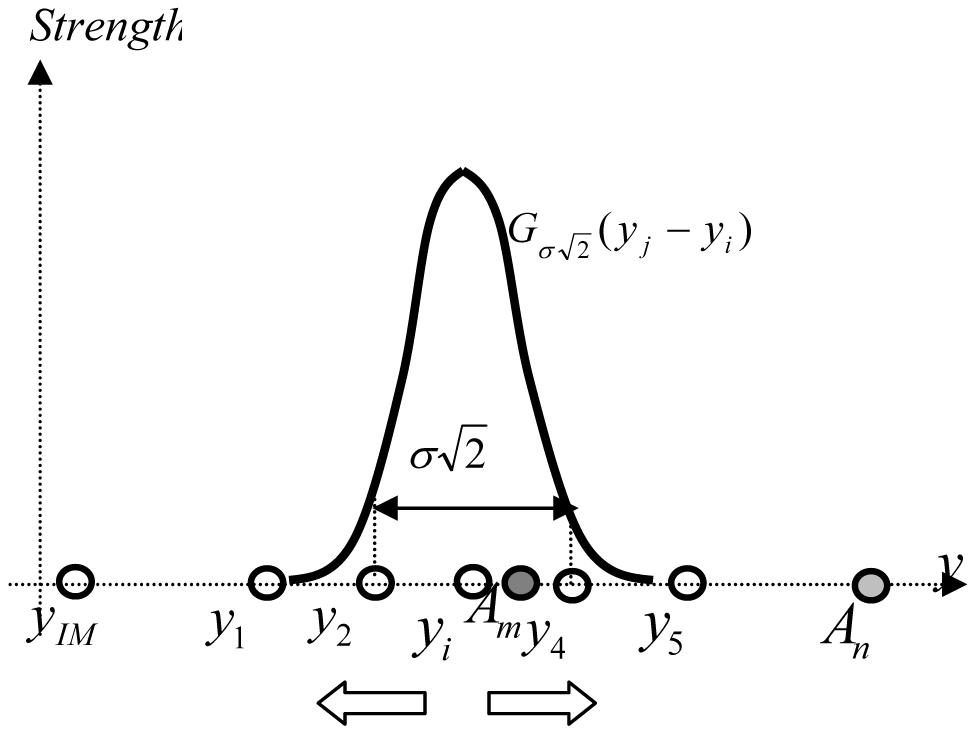Here we notice two problems. One is that samples $y_{1}$ and $y_{5}$ also need to be included within the range for the search, but the impulsive noise\textendash{}corrupted $y_{IM}$ should not be. This means the scope of influence must be expanded\textemdash{}but not too much.

Another problem is that the strong repelling force among the output samples needs to be reduced when they are near their own target,$A_{m}$. These two tasks of broadening the range and reducing the force can be carried out by modifying kernel size $\sigma \sqrt{2}$ towards a larger value, but not too large, as depicted in Fig. 2. The increased kernel size (solid line) covers $y_{1}$ and $y_{5}$, but not $y_{IM}$, and reduces the strength.

For the output samples near their own target $A_{m}$, we can bring them closer to $A_{m}$ by applying the approach to $IP(d,y)$ in (5). In this case, $IP(d,y)$ is maximized as cost function $C_{MED2}$ is minimized, so the gap distance between $A_{m}$ and $y_{i}$ located within the range of $\sigma$ decreases according to the maximization of $IP(d,y)$.

In Fig. 3, we can increase the force, decreasing kernel size $\sigma$ of $G_{\sigma }(A_{m}-y_{i})$ in$IP(d,y)$, as indicated by the solid line. Then, the two samples, $y_{3}$ and $y_{4}$, within the modified kernel size move closer together towards their target, $A_{m}$. However contracting the scope of influence (the solid line) leads to discarding $y_{1}$ and $y_{5}$ located relatively close to $A_{m}$. They can wander around, not being within the scope of influence of $IP(d,y)$. This task leads us to modify kernel size $\sigma$ of $IP(d,y)$ towards a smaller value (but not too small).

From analysis of the scope of information potentials $IP(y,y)$ and $IP(d,y)$, we can develop many possible approaches to manipulating scope to obtain better performance. As one of the simple methods, we propose a modified cost function, $C_{\textit{proposed}}(\alpha ,\beta )$, as follows:

##### (11)
$C_{\textit{proposed}}(\alpha ,\beta )=IP_{\alpha }(y,y)-2\cdot IP_{\beta }(d,y)$,

where

##### (12)
$IP_{\alpha }(y,y)=\frac{1}{N^{2}}\sum _{i=1}^{N}\sum _{j=1}^{N}G_{\alpha \sigma \sqrt{2}}(y_{j}-y_{i})$,
##### (13)
$IP_{\beta }(d,y)=\frac{1}{M}\frac{1}{N}\sum _{m=1}^{M}\sum _{i=1}^{N}G_{\beta \sigma }(A_{m}-y_{i})$.

With this proposed cost function, $C_{\textit{proposed}}(\alpha ,\beta )$, a new blind algorithm for recursiveweightadjustment can be derived as follows:

##### (14)
$\mathbf{W}_{k+1}^{F}=\mathbf{W}_{k}^{F}-$$\frac{\mu }{2N^{2}\alpha ^{2}\sigma ^{2}}\sum _{i=k-N+1,}^{k}\sum _{j=k-N+1}^{k}(y_{j}-y_{i})$$\cdot G_{\alpha \sigma \sqrt{2}}(y_{j}-y_{i})\cdot (\mathbf{X}_{i}-\mathbf{X}_{j})$$-\frac{2\mu }{MN\beta ^{2}\sigma ^{2}}\sum _{i=k-N+1,}^{k}\sum _{m=1}^{M}(A_{m}-y_{i})$$\cdot G_{\beta \sigma }(A_{m}-y_{i})\cdot \mathbf{X}_{i}$,
$\mathbf{W}_{k+1}^{B}=\mathbf{W}_{k}^{B}-$$\frac{\mu }{2N^{2}\alpha ^{2}\sigma ^{2}}\sum _{i=k-N+1,}^{k}\sum _{j=k-N+1}^{k}(y_{j}-y_{i})$$ \cdot G_{\alpha \sigma \sqrt{2}}\left(y_{j}-y_{i}\right) \cdot\left(\hat{\mathbf{D}}_{i-1}-\hat{\mathbf{D}}_{j-1}\right) $$-\frac{2\mu }{MN\beta ^{2}\sigma ^{2}}\sum _{i=k-N+1,}^{k}\sum _{m=1}^{M}(A_{m}-y_{i})\cdot G_{\beta \sigma }(A_{m}-y_{i})\cdot \hat{\mathbf{D}}_{i-1}. ## 4. Results and Discussion For simplicity, we consider the baseband-equivalent data transmission system in Fig. 4, with transmitted data d_{k} at sampletime k selected from among symbol points \{A_{1}=-3,A_{2}=-1,A_{3}=1,A_{4}=3\}, multi-path channel H(z), received signal x_{k}, and equalizer outputy_{k}. The feed-forward filter is P=7, and the feedback filter has Q=4 weights. Channel model H(z) is from underwater channel data actually acquired from a shallow-water communications experiments, described in z-transform  as follows: Table 1. Parameter values for minimum MSE.  α β Minimum MSE (dB) 3.0 0.8 -25.5 0.9 -27.8 1.0 -27.8 4.0 0.8 -26.4 0.9 -28.7 1.0 -27.7 5.0 0.8 -22.1 0.9 -27.8 1.0 -25.7 ##### (16) H\left(z\right)=0.798z^{-4}+0.543z^{-6}+0.259z^{-8} The impulsive noise model composed of background additive white Gaussian noise (AWGN) and impulse noise (IM) is the same as the one used in . The distribution of impulsive noise nis ##### (17)$$ f(n)=(1-\varepsilon) / \sigma_{A W G N} \sqrt{2 \pi} \cdot \exp \left[-n^{2} / 2 \sigma_{A W G N}^{2}\right]$+\varepsilon /\sqrt{2\pi (\sigma _{AWGN}^{2}+\sigma _{IM}^{2})}\cdot \exp [-n^{2}/2(\sigma _{AWGN}^{2}+\sigma _{IM}^{2})]$where$\varepsilon =0.03$,$\sigma _{IM}^{2}=50$, and$\sigma _{AWGN}^{2}=0.001$is the variance of background AWGN. The step-size was set to$\mu =0.01$, and data-block size$N$for kernel estimation was set to$10$. Kernel size$\sigma $was$0.8$. All these parameters were chosen to show the lowest steady state MSE values. The lowest steady state MSE values of the proposed algorithm in (14) and (15) are depicted in Table 1 for various values of$\alpha $in$IP_{\alpha }(y,y)$and of$\beta $in$IP_{\beta }(d,y)$. In addition to Table 1, steady state learning curves are shown in Figs. 5 and 6 for the optimum$\beta $= 0.9 and$\alpha $=4.0, respectively. We determined that the scope of influence for$IP(y,y)$should be expanded (but not too much), and that$IP(d,y)$should be contracted, but again, not too much. This result is in accordance with the analysis in Section 3. With optimum parameters$\alpha $=4.0 and$\beta $=0.9 for the proposed algorithm, we show the performance enhancement above MED2 with DF (without scope modification) in Figs. 7 and 8 for MSE convergence and error distribution, respectively. Through a comparison of MSE convergence and error distribution that reveals how frequently each system error value occurs, we show the performance difference between the proposed algorithm depicted in (14) and (15), and MED2 with DF in (9) and (10), as done in . In the results shown in Fig. 7, the performance gain from scope modification is about 10 dB or more. We see the difference more obviously in the error probability distribution in Fig. 8, showing a sharper bell-shaped probability density for error samples concentrated around zero than MED2 with DF. Fig. 5. Steady state learning curves for various values of$\alpha $, when$\beta $= 0.9.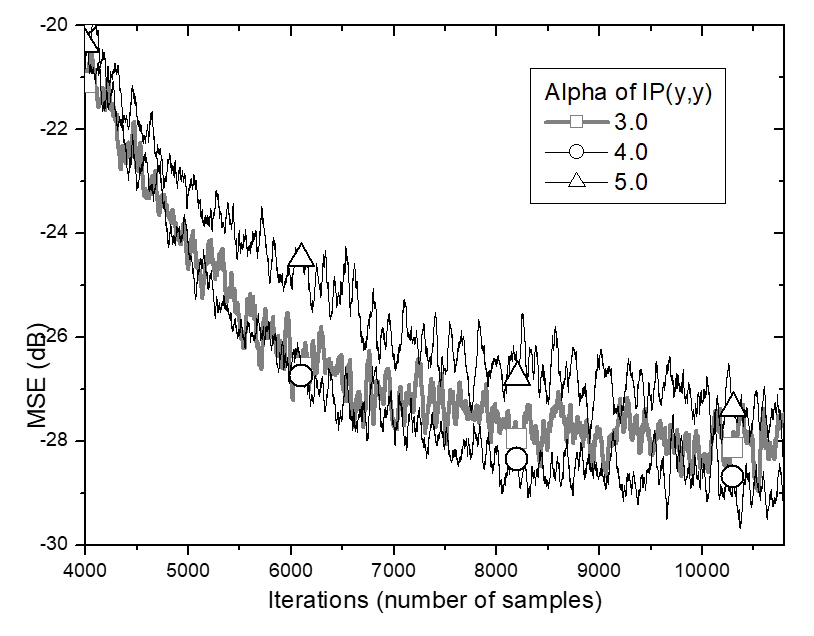Fig. 6. Steady state learning curves for various values of$\beta $when$\alpha $=4.0.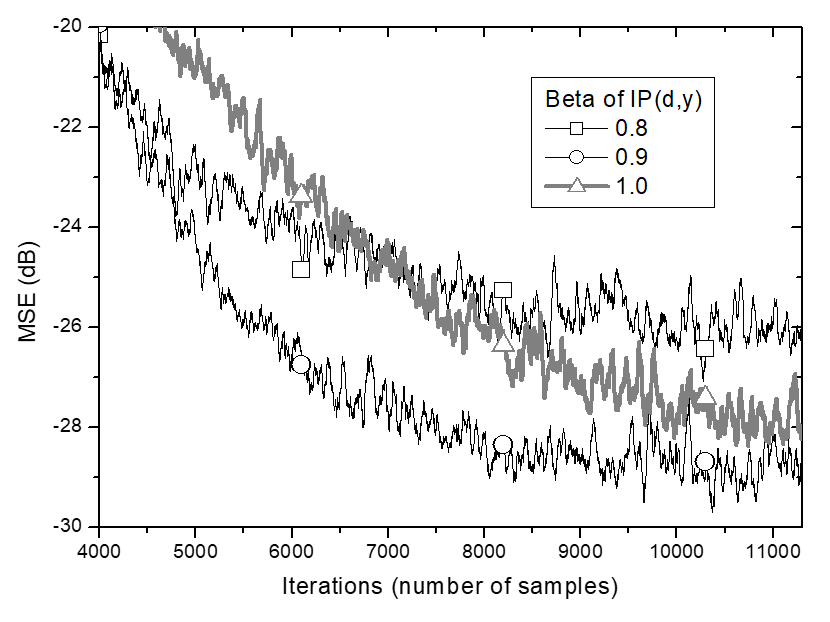Fig. 7. MSE performance comparison for the underwater channel.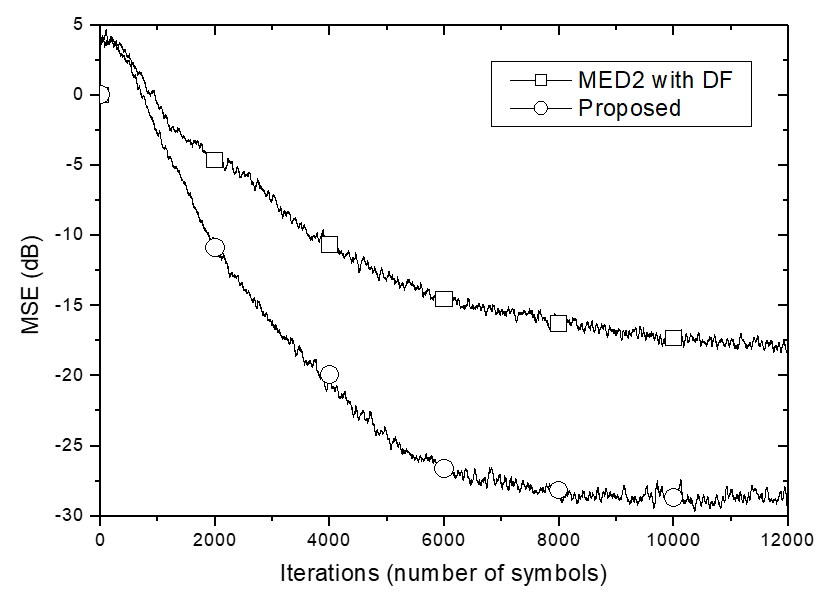Fig. 8. Error distribution comparison for the underwater channel.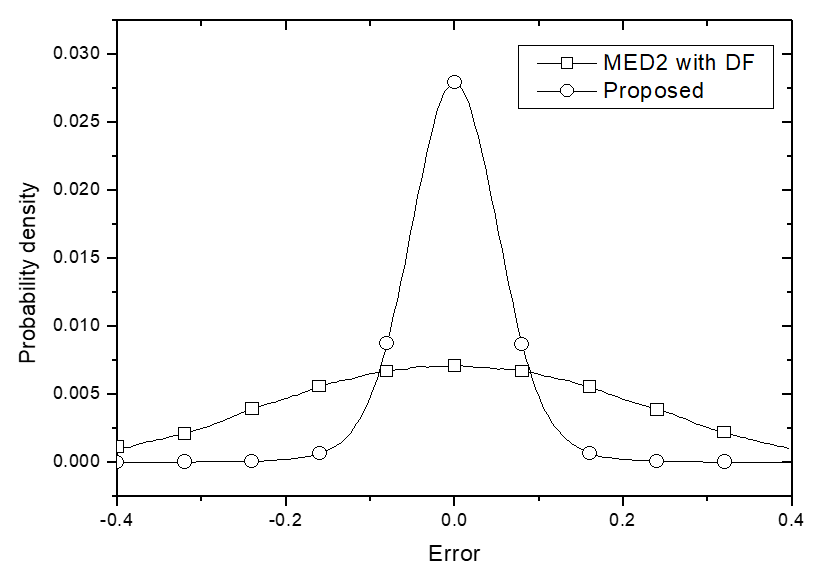## 5. Conclusion To cope with impulsive noise and severe channel distortions more effectively in blind DFE systems, a modification approach to the scope of influence of information potentials was presented in this paper. The scope of information potential for output samples can be expanded for wide searching for their own targets, but not too much, because large samples corrupted by impulsive noise should not be included in the scope, and the repelling force among the output samples near their own targets needs to be reduced. By contrast, the scope of information potential for symbol point and output pairs can be contracted for the output samples within the range to obtain a stronger force to concentrate on their target, but not too much in order not tolose relatively closely located output samples. According to the analysis, a modified cost function employing optimum ranges of information potentials is proposed, and a related DFE algorithm is derived. The simulation results carried out in the environment of a severe multipath channel, obtained from shallow-water communication experiments and impulsive noise, show that optimum ranges of information potentials for channel and noise are in accordance with the analysis, and the MSE performance gain from scope modification is above 10 dB. These simulation results and their analysis lead us to the conclusion that the scope of IP influence should be applied separately according to the roles of IP under impulsive noise, and that the proposed method is significantly robust against strong impulsive noise, and is superior when compensating for ISI from severe channel distortion. ### ACKNOWLEDGMENTS ### REFERENCES 1 Proakis J., 1989, Digital Communications, McGraw-Hill 2$^{\mathrm{nd}}$ed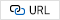2 Yabuuchi Y., Umehara D., Morikura M., Hisada T., Ishico S., Horihata S., 2010, Measurement and analysis of impulsive noise on in-vehicle power lines, In Proceedings of ISPLC'10, pp. 325-3303 Daifeng Z., Tianshuang Q., 2006, Underwater sources location in non-Gaussian impulsive noise environments, Digital Signal Processing, Vol. 16, pp. 149-1634 Kim N., Byun H., Kweon K., 2012, Decision feedback approach to blind algorithms in impulsive noise, In Proc. of the 35$^{th}\$ Telecommunications and Signal Processing Conference. Prague (Czech), pp. 653-6575
Principe J., Xu D., Fisher J., 2000, Information theoretic learning, in: Haykin, S., Unsupervised Adaptive Filtering, Wiley, New York.6
Parzen E., 1962, On the estimation of a probability density function and the mode, Ann. Math. Stat., Vol. 33, pp. 10657
Viola P., Schraudolph N., Sejnowski T., 1995, Empirical entropy manipulation for real-world problems, In Proc. of the NIPS 8 (Neural Infor. Proc. Sys.) Conference, pp. 851-8578
Reny , 1976, On measures of entropy and information, Selected papers of Alfred Renyi. Akademia Kiado, Budapest.9
Kim S., Youn C., Lim Y., 2010, Performance analysis of receiver for underwater acoustic communications using acquisition data in shallow water, Journal of Acoustical Society of Korea, Vol. 29, No. 5, pp. 303-313## Author

Namyong Kim received the B. S., M. S. and Ph. D degree from Yonsei University, all in electronic engi-neering in 1986, 1988 and 1991, respectively. From 1992 to 1997 he was with Catholic Kwandong University, Korea. Currently he serves as a professor at School of Electronics, Information & Communication Engineering, Kangwon National University, Korea. His research interests are in~adaptive signal processing in mobile communications and information theoretic learning (ITL) algorithms.

Kihyeon Kwon is Professor of Electronic, Information and Communi-cations Engineering at the Kangwon National University (KNU), Korea. He received his B.S., M.S. and Ph.D. degrees in Computer Science from Kangwon National University, Korea, in 1993, 1995 and 2000, respectively. He has served on an Editor of the Journal of Digital Contents Society. His research interests include communication systems, blind type algorithms and data fusion recognition.